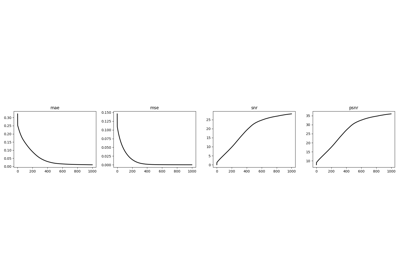# pylops.optimization.cls_sparsity.FISTA#

class pylops.optimization.cls_sparsity.FISTA(Op, callbacks=None)[source]#

Fast Iterative Shrinkage-Thresholding Algorithm (FISTA).

Solve an optimization problem with $$L_p, \; p=0, 0.5, 1$$ regularization, given the operator Op and data y. The operator can be real or complex, and should ideally be either square $$N=M$$ or underdetermined $$N<M$$.

Parameters
Oppylops.LinearOperator

Operator to invert

Raises
NotImplementedError

If threshkind is different from hard, soft, half, soft-percentile, or half-percentile

ValueError

If perc=None when threshkind is soft-percentile or half-percentile

OMP

Orthogonal Matching Pursuit (OMP).

ISTA

Iterative Shrinkage-Thresholding Algorithm (ISTA).

SPGL1

Spectral Projected-Gradient for L1 norm (SPGL1).

SplitBregman

Split Bregman for mixed L2-L1 norms.

Notes

Solves the following synthesis problem for the operator $$\mathbf{Op}$$ and the data $$\mathbf{y}$$:

$J = \|\mathbf{y} - \mathbf{Op}\,\mathbf{x}\|_2^2 + \epsilon \|\mathbf{x}\|_p$

or the analysis problem:

$J = \|\mathbf{y} - \mathbf{Op}\,\mathbf{x}\|_2^2 + \epsilon \|\mathbf{SOp}^H\,\mathbf{x}\|_p$

if SOp is provided.

The Fast Iterative Shrinkage-Thresholding Algorithm (FISTA)  is used, where $$p=0, 0.5, 1$$. This is a modified version of ISTA solver with improved convergence properties and limited additional computational cost. Similarly to the ISTA solver, the choice of the thresholding algorithm to apply at every iteration is based on the choice of $$p$$.

1

Beck, A., and Teboulle, M., “A Fast Iterative Shrinkage-Thresholding Algorithm for Linear Inverse Problems”, SIAM Journal on Imaging Sciences, vol. 2, pp. 183-202. 2009.

Methods

 __init__(Op[, callbacks]) callback(x, *args, **kwargs) Callback routine finalize([show]) Finalize solver run(x[, niter, show, itershow]) Run solver setup(y[, x0, niter, SOp, eps, alpha, ...]) Setup solver solve(y[, x0, niter, SOp, eps, alpha, ...]) Run entire solver step(x, z[, show]) Run one step of solver

## Examples using pylops.optimization.cls_sparsity.FISTA#